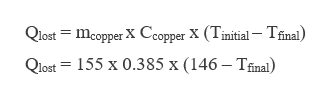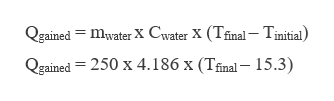A 155.0 g piece of copper at 146 oC is dropped into 250.0 g of water at 15.3 oC. (The specific heat of copper is 0.385 J/goC.) Calculate the final temperature of the mixture. (Assume no heat loss to the surroundings.)

Question

A 155.0 g piece of copper at 146 oC is dropped into 250.0 g of water at 15.3 oC. (The specific heat of copper is 0.385 J/goC.) Calculate the final temperature of the mixture. (Assume no heat loss to the surroundings.)

Step 1

The heat lost by copper will be equal to the heat gained by water as there is no loss of heat to the surrounding. The final temperature of the mixture can be calculated as,

Step 2

The heat lost by copper having a mass of 155 g is calculated as,help_outlineImage TranscriptioncloseQlost mcopper X Ccopper X (Tinitial- Tfinal) Qlost 155 x 0.385 x (146 - Tfinal) fullscreen
Step 3

The heat gained by water having a mass of 250 g and specific hea...help_outlineImage TranscriptioncloseQgained mwater X Cwater X (Tfinal- Tinitial) Qgained 250 x 4.186 x (Tfinal- 15.3) fullscreen

Want to see the full answer?

See Solution

Want to see this answer and more?

Our solutions are written by experts, many with advanced degrees, and available 24/7

See Solution
Tagged in

Chemical Thermodynamics